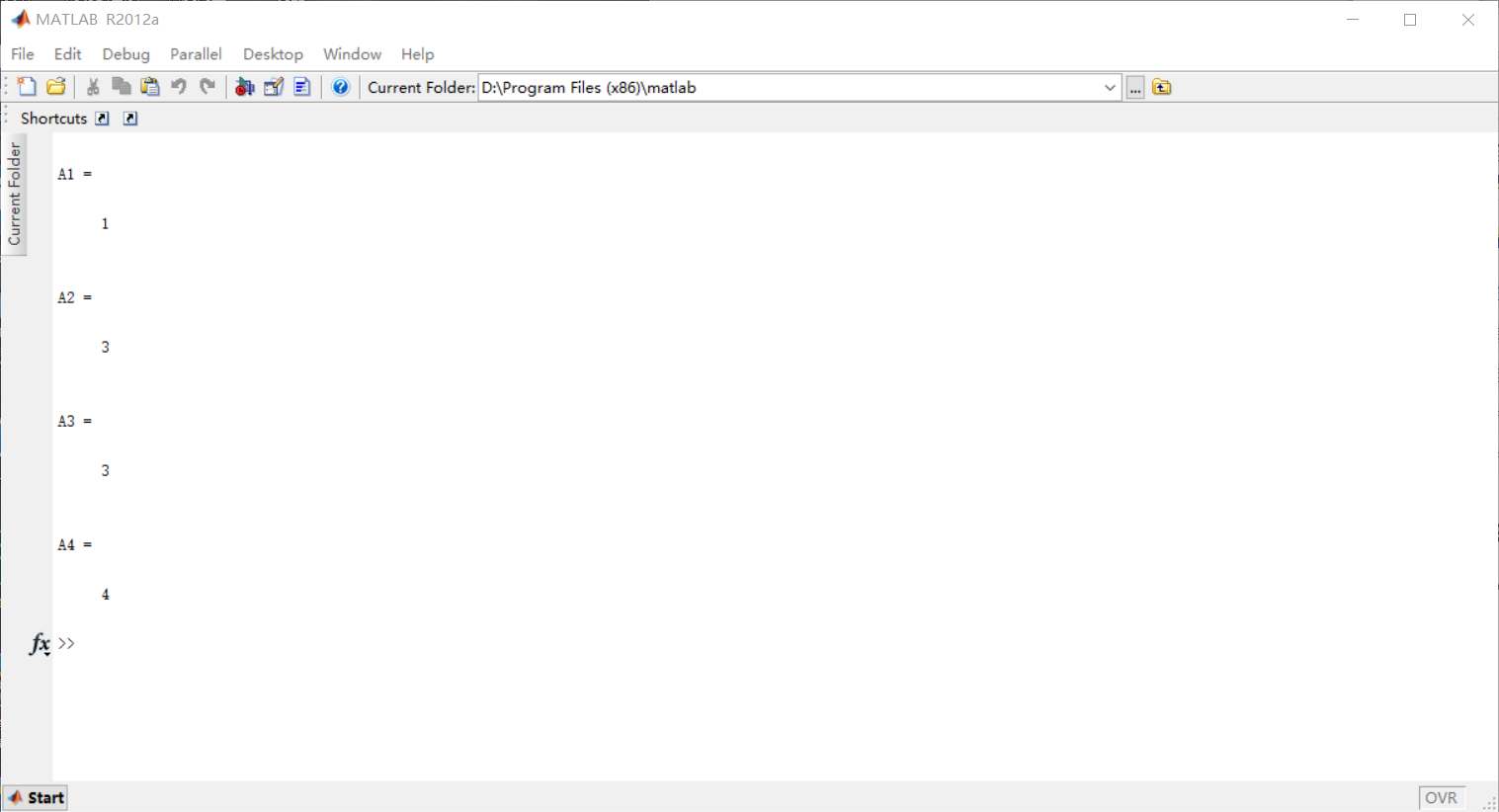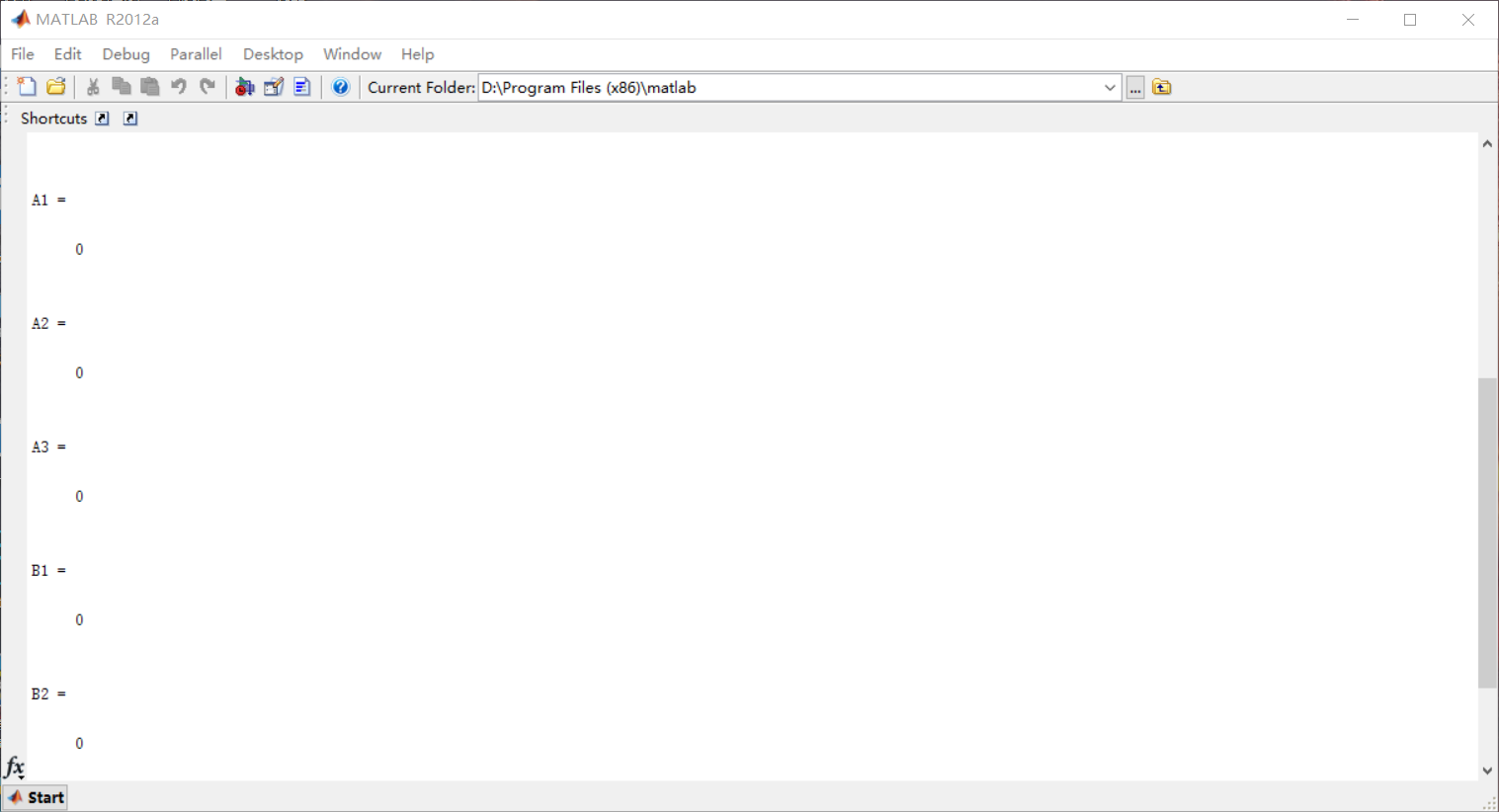# Matlab矩阵元素的处理

1. 矩阵元素的取整
2. 矩阵元素的取模和取余

clear all;
clc
A1 = floor(1.11);
A2 = ceil(2.22);
A3 = round(3.33);
A4 = fix(4.44);A1 = mod(10,5), A2 = mod(10,-5), A3 = mod(-10,5)
B1 = rem(10,5), B2 = rem(10,-5), B3 = rem(-10,5)©️2019 CSDN 皮肤主题: 数字20 设计师: CSDN官方博客